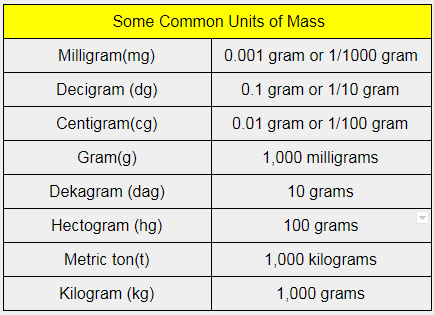Checkout JEE MAINS 2022 Question Paper Analysis : Checkout JEE MAINS 2022 Question Paper Analysis :

# Unit of Mass

Before understanding the unit of mass it is very crucial for students to know what is mass. In simple terms, mass is the quantity of matter in an object. More significantly, it can be both a property of a physical body and a measure of its resistance to acceleration when some amount of force is applied. However, one important point students should remember here is that mass is different from the weight even though the terms are sometimes used interchangeably. Also, they have different units. Let’s look at the SI unit for mass and other units of mass in this article.

## What Is Mass?

Mass is defined as the matter that is present in the given object. Following are the most commonly used units for the measurement of mass:

• Gram
• Kilogram

## SI Unit of Mass

The standard SI unit of mass is as:

 kilogram (kg)

### Other Units of Mass

Other units that are accepted as the units of mass are gram (g) and its multiples and submultiples, a tonne (t) (or “metric ton”), electronvolt (eV), the atomic mass unit (u) which is most convenient for expressing the masses of atoms and molecules.Related articles:

### What is the difference between mass and weight?

The difference between mass and weight is that mass is the quantity of matter that is contained in an object. It is a constant quantity measured in kilograms and is a scalar quantity.
Whereas weight is the force with which an object gets pulled towards the earth. It is not a constant quantity, measured in Newton and is a vector quantity.

### The modern concept of mass was introduced by whom?

The modern concept of mass was introduced by Isaac Newton.

### What do derived units mean? Give examples?

Derived units are obtained from the fundamental units and are the products and ratios of the fundamental units. Units of velocity and acceleration are examples of derived units.

### What do supplementary units mean?

The dimensionless quantities that are used for the derivation of derived physical quantities along with fundamental units are known as supplementary units.

### What does a coherent system of units mean?

The system of units which has derived units obtained from the base units without any numerical factor other than 1 is the coherent system of units. 1 Watt is an example of a coherent system of units as it is a ratio of 1 Joule to 1 second.

Hope you have understood the SI unit for mass, and other units of mass in this article. Stay tuned with BYJU’S and fall in love with learning.Test your Knowledge on unit of magnetic flux